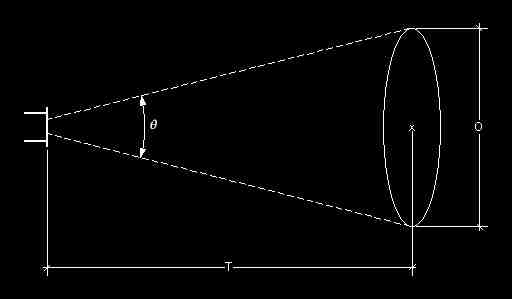# Robin Barton

Glossary

### Lighting

Beam Angle Calculator
DMX Calculator
Fixture Database
Lamp Database

# Beam Calculator

The tools on this page allow you to calculate various characteristics of a beam of light depending on what you already know. The terms used are:

 Beam Angle (θ) The optical dispersion of the light beam - the angle at which the light spreads. This is often shown on the lens tube (e.g. 26°) and, in the case of zoom profiles is a range (e.g. 18° - 32°). Beam Diameter (O) The diameter of the beam of light at the throw distance from the source. Throw (T) The distance between the front of the luminaire and the surface being lit. It is the measurement you would get if you put a tape measure from the centre of the lense down the centre of the beam to the surface being lit.

## Basic Calculations

This section assumes that the beam of light hits a surface staight on, i.e. the beam is circular not elliptical. For other instances, see the "Advanced Calculations" setcion below;I know the beam angle and throw:I know the beam diameter and throw: Beam Angle (θ) ° Beam Diameter (O) m Throw (T) m Throw (T) m Beam Diameter (O) m Beam Angle (θ) °